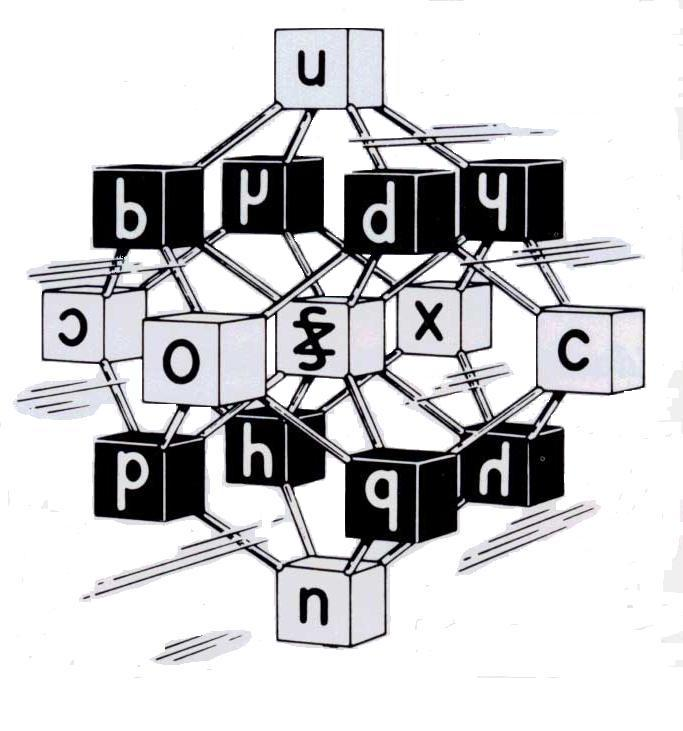Miracles of the Qur'an# WHAT KIND OF LOGIC DO YOU HAVE?

Do not forget that this probability is the result of a single table. If we calculated the probability of all the phenomena related to 19 in the Quran, the number would be astronomical. This probability is much smaller than finding one single grain of sand after hiding it somewhere in the world. And it is even smaller than finding a piece of an atom randomly, after hiding it in a galaxy somewhere in the universe. The number of particles of the atoms in the universe is thought to be 1080. If we brought all the tables related to 19 together, the probability of forming a mathematical pattern coincidentally like the one we have would be even smaller than 1/1080.

We wonder what kind of logic you might have. Do you think that the Prophet was the first inventor of the computer, or that he was hiding a computer in the sand dunes of the desert in order to perform a miracle like this? Or do you think that we should accept a probability smaller than one over the total of all sub-atomic particles in the universe in order to ignore the Quran’s miracle? Or will you accept this great miracle of the Quran and appreciate that all humans and jinns could not compose anything like it, even if they worked together? What kind of logic do you possess? What kind of logic would you prefer to possess?

88- Say, “If all the humans and the jinns came together in order to produce a Quran like this, they would surely fail, no matter how much assistance they lent one another.”
17-The Children of Israel, 88